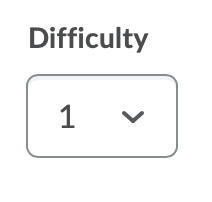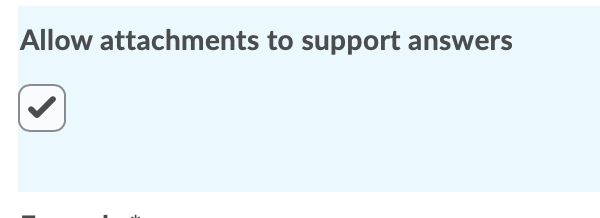# Create Arithmetic Questions in the Question Library

Arithmetic questions enable instructors to assess students’ knowledge and comprehension of mathematics and number theory. Instructors can ensure each respondent receives a unique question by including variables enclosed with curly braces that randomly generate numbers within the problem.

For example, if you set variables x, y, and z with a Min 1 to Max 5 number range in 1-step increments, the question “You have {x} green marbles, {y} red marbles, and {z} blue marbles. How many marbles do you have in total?” will randomly generate a rational number (1, 2, 3, 4, 5) for {x}, {y}, and {z}.

As a best practice, it is recommended that you create written response (WR) question types for arithmetic problems that require students to demonstrate their calculations and show their work.

## Understanding Arithmetic and Significant Figures Question Components

In arithmetic questions, use answer precision to limit the number of acceptable decimal places allowed in a response. You can require that correct answers contain a specific number of decimal places.

Use tolerance levels to accept near-accurate, estimated, or rounded answers.

Watch how to create arithmetic questions

Note: For a secure and consistent editing experience, JavaScript and non-standard HTML will automatically be filtered out. If your existing questions contain JavaScript or non-standard HTML, the questions continue to display as intended until an instructor edits and saves the quizzes, which removes the JavaScript and non-standard HTML.

1. On the nav bar, select the Activities menu, then click either Quizzes, Surveys, or Self Assessments. In the example below, we selected Quizzes.2. From the tool navigation, click Question Library.3. Select New.4. Select the question type:  Arithmetic Question (2+2).
5. Optional: Title Note: We recommend leaving the Title field blank. The Question Text will become the title by default, allowing you to see the question without having to click to open it.6. Required: Points7. Optional: Difficulty Level8. Required: Question Text:  Enclose all variables with curly braces if you want to generate random numbers. To display the value of a variable, ensure that the variable is enclosed in curly braces. For example:What is the sum of {x} and {y}?
Note: The Graphical Equation editor can be especially helpful if the question contains a complicated formula. If you are working on a device with a small screen, you may have to click the ellipses for the Graphical Equation menu to appear.9. Optional: Insert an Image and Alternative Text.10. Optional: Allow attachments to support answers:  Check the box to allow students to attach files with evidence supporting their answer.• When students are reviewing their quiz submissions, if they are allowed to view their quiz responses, they can view their uploaded attachments.
• The maximum file size for a single file or embedded image attached to a quiz response is set to 102400 KB (100MB). The maximum file size for all files attached or embedded in a single quiz question response is set to 102400 KB (100MB).
11. Enter the solution’s formula, setting variables inside curly braces. See Types of Supported Enumerations in the Formula Field.12. To verify your formula before sharing it with students, click Test.
13. Use the Answer Precision field to define the number of decimal places the answer may contain; check Enforce Precision if you want to require this number of decimal places in the correct answer. Note: If you enforce precision, we suggest specifying the number of decimal places a correct answer should contain in your question or in the quiz instructions. Learn about Understanding Quiz Answer Rounding Rules.14. To accept near-accurate, estimated, and/or rounded answers, enter a Tolerance level:
15. Use this option if the correct answer should include a unit of measurement (mm, cm, grams, inches, etc.):
16. Select the Evaluation Option. There are three possible grading options:
• Text/Case Insensitive – Auto-grading searches for a matching character pattern in the answer text with or without letter case correctness.
• Case-Sensitive – Auto-grading searches for a matching character pattern in the answer text that must have letter case correctness. Proper capitalization is required.
• Regular Expression – Auto-grading uses meta-characters to search for one or more matching strings in the answer text’s character pattern. What you set as meta-character parameters helps determine letter case sensitivity. Learn more about Regular Expressions, see Understanding Regular Expressions.
17. In the Variables area, do the following:
18. Optional: Add or remove Variables, as needed, to match the number of variables in the formula. Add a variable by clicking the Add Variable link. Delete a variable by selecting the Remove Variable (trash can icon).
19. Optional: To add overall feedback for the question, select Add Feedback. Learn about Quiz Question Feedback.
20. Optional: To add a hint to the question, select Add Hint. Learn about Quiz Question Hints.